Governing equations and boundary conditions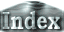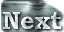The governing equations are those of a two-dimensional Boussinesq fluid,(1)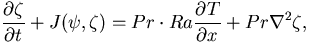(2)

where T is temperature,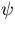is stream function,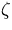is vorticity, Pr is the Prandtl number, which is the ratio of viscosity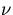and thermal conductivity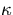. Ra is the Rayleigh number, which describes the unstable extent of the stratification. J(A,B) is the Jacobian. These equations are non-dimensionalized using the thickness of the fluidlayer as the length scale, the thermal diffusion time as the time scale, and temperature difference between the top and bottom boundaries as the temperature scale.

The thermal boundary conditions correspond to fixed temperatures at the top and the bottom boundaries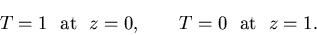(3)
The kinematic and dynamic boundary conditions are impermeable, and free-slip: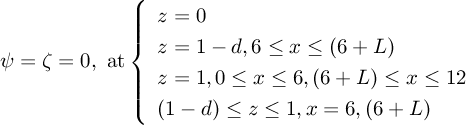(4)
The horizontal boundaries are periodic.

Further explanations of these equations and the boundary conditions are given in Appendix A.

 Governing equations and boundary conditions